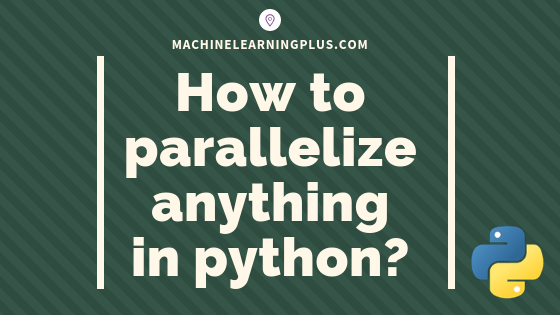## Parallel Processing in Python – A Practical Guide with Examples

Parallel processing is a mode of operation where the task is executed simultaneously in multiple processors in the same computer. It is meant to reduce the overall processing time. In this tutorial, you’ll understand the procedure to parallelize any typical logic using python’s multiprocessing module. 1. Introduction Parallel processing is a mode of operation where …